# 海洋脐带缆铠装钢丝的结构设计Structural Design of Marine Umbilical Armored Wire

• 全文下载: PDF(843KB)    PP.223-230   DOI: 10.12677/MET.2019.83028
• 下载量: 187  浏览量: 642

Armored steel wire is an important strength component of the umbilical, which can bear the load of stretching and torsion in complex marine environment and can protect the central core. In order to ensure the torsion balance and stress balance of umbilical, the armored steel wire is usually designed as two or more layers and wound outside the central core in opposite directions. In this paper, a theoretical design method of armored steel wire of umbilical is discussed, and a theoretical model of double armored umbilical is established. The results show that the spiral angle and diameter of the armored wire have a certain effect on the mechanical strength of the umbilical.

1. 引言

2. 脐带缆铠装钢丝尺寸设计

1) 平截面假定，即变形前后螺旋铠装钢丝截面保持平整，伸长和扭转等变形量相等。

2) 小变形假定，即在线性分析过程中，管缆的应变参数要远小于1。

3) 轴向构件和螺旋构件材质均符合均匀、各向同性和线弹性的假定。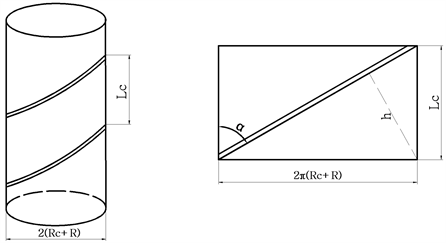Figure 1. Schematic diagram of helical wirealong the central cylinder

${n}_{内}\cdot 2R/\mathrm{sin}\alpha \le {L}_{c}=2\text{π}\left({R}_{c}+R\right)/\mathrm{tan}\alpha$

${n}_{内}\le \frac{\text{π}\left({R}_{\text{c}}+R\right)\mathrm{cos}\alpha }{R}$ (1)

${n}_{外}\le \frac{\text{π}\left({R}_{\text{c}}+3R\right)\mathrm{cos}\alpha }{R}$ (2)

${n}_{内}$${n}_{外}$ 应取整数。

3. 脐带缆铠装钢丝的强度设计

${k}_{11}=\underset{i=1}{\overset{n}{\sum }}\left[{E}_{i}{A}_{i}\left(1-\frac{\Theta }{2}\frac{{R}_{c}}{{R}_{i}}{\mathrm{tan}}^{2}{\alpha }_{i}\right){\mathrm{cos}}^{3}{\alpha }_{i}\right]+{E}_{c}{A}_{c}$ (7)

${k}_{12}=\underset{i=1}{\overset{n}{\sum }}{E}_{i}{A}_{i}{R}_{i}\mathrm{sin}{\alpha }_{i}{\mathrm{cos}}^{2}{\alpha }_{i}$ (8)(9)

${k}_{22}=\underset{i=1}{\overset{n}{\sum }}{E}_{i}{A}_{i}{R}_{i}^{2}{\mathrm{sin}}^{2}{\alpha }_{i}\mathrm{cos}{\alpha }_{i}+{G}_{c}{J}_{c}$ (10)

3.1. 铠装脐带缆理论分析模型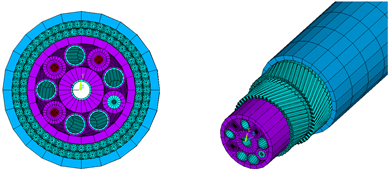Figure 2. Model of double armoured umbilicalTable 1. Dimension of umbilical central core

3.2. 铠装钢丝螺旋角度的设计Table 3. Number of armoured steel wire

3.2.1. 铠装钢丝螺旋角度与整体拉伸刚度的关系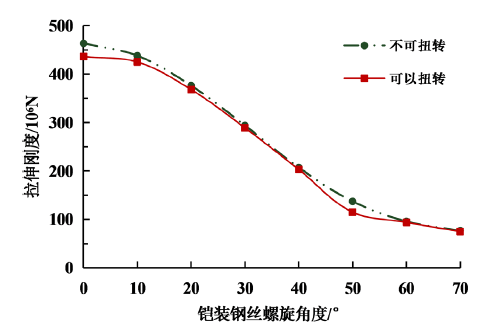Figure 3. Relationship between torsional stiffness of umbilical and spiral angel of armored steel wireand spiral angel of armored steel wire

3.2.2. 铠装钢丝螺旋角度与整体扭转刚度的关系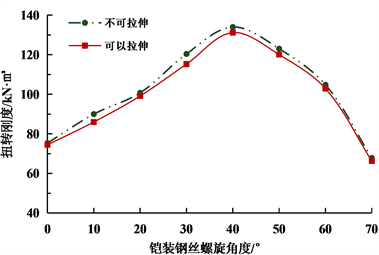Figure 4. Relationship between torsional stiffness of umbilical and spiral angel of armored steel wireand spiral angel of armored steel wire

3.3. 铠装钢丝半径的设计Table 4. Number of armoured steel wire

3.3.1. 铠装钢丝半径与整体拉伸刚度的关系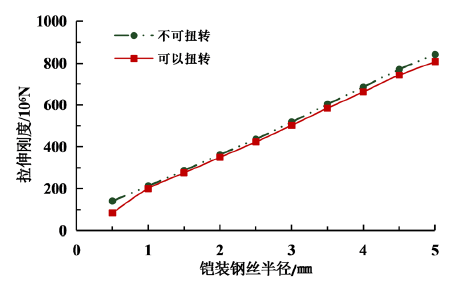Figure 5. Relationship between tensile stiffness of umbilical and radius of armored steel wireand radius of armored steel wire

3.3.2. 铠装钢丝半径与整体扭转刚度的关系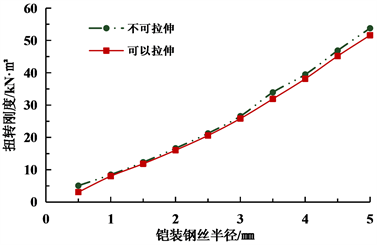Figure 6. Relationship between torsional stiffness of umbilical and radius of armored steel wireand radius of armored steel wire

4. 结论

1) 对脐带缆铠装钢丝初步设计时，由中心内核半径、铠装钢丝半径和螺旋角度即可初步确定各层铠装钢丝的数量。

2) 在两种约束条件下，脐带缆模型的拉伸刚度随着铠装钢丝螺旋角度的增大而减小，螺旋角度较小时，拉伸刚度削弱不明显，螺旋角度较大时，拉伸刚度急剧下降。脐带缆扭转刚度随着铠装钢丝螺旋角度的增大先缓慢增大而后迅速下降。

3) 在两种约束条件下，脐带缆模型的拉伸刚度和扭转刚度均随着铠装钢丝半径的增大而增大，且近似为线性关系。工程中可在满足外径重量比的前提下，合理选用半径较大的铠装钢丝。

NOTES

*通讯作者。

  李志刚, 贾鹏, 王洪海, 张宁, 王立权. 水下生产系统发展现状和研究热点[J]. 哈尔滨工程大学学报, 1-8.  王玮, 孙丽萍, 白勇. 水下油气生产系统[J]. 中国海洋平台, 2009, 24(6): 41-45.  Yue, Q.J., Lu, Q.Z., Yan, J. and Zheng, J.X. (2013) Andrew Palmer. Tension Behavior Prediction of Flexible Pipelines in Shallow Water. Ocean Engineering, 58, 201-207. https://doi.org/10.1016/j.oceaneng.2012.11.002  Hruska, F.H. (1951) Calculation of Stresses in Wire Ropes. Wire and Wire Products, 26, 766-767, 799-801.  Hruska, F.H. (1952) Radial Forces in Wire Ropes. Wire and Wire Products, 27, 459-463.  Knapp, R.H. (1979) Derivation of a New Stiffness Matrix for Helically Armoured Cables Considering Tension and Torsion. International Journal for Numerical Methods in Engineering, 14, 515-529. https://doi.org/10.1002/nme.1620140405  Knapp, R.H. (1981) Torque and Stress Balance Design for Helically Armored Cables. Journal of Engineering for Industry, 103, 61-66. https://doi.org/10.1115/1.3184460  周学军, 王瑛剑, 伍先俊, 等. 一种精确海缆铠装钢丝几何建模法探讨[J]. 武汉理工大学学报, 2009, 31(15): 80-83.  杨志勋. 脐带缆结构设计与分析方法研究[D]: [硕士学位论文]. 大连: 大连理工大学, 2012: 33-35.  张梁, 丁伟宸, 徐霄龙, 等. 脐带缆铠装钢丝力学性能研究[J]. 中国造船, 2015(56): 42-51.  Custo dio, A.B. and Vaz, M.A. (2002) A Nonlinear Formulation for the Axisym-Metric Response of Umbilical Cables and Flexible Pipes. Applied Ocean Research, 24, 21-29. https://doi.org/10.1016/S0141-1187(02)00007-X